﻿ 计量系统下基于边缘计算的电量预测 Electricity Forecasting Based on Edge Computing under Metering System

Smart Grid
Vol. 09  No. 01 ( 2019 ), Article ID: 28981 , 8 pages
10.12677/SG.2019.91003

Electricity Forecasting Based on Edge Computing under Metering System

Jun Yang1, Aidong Xu2, Wenjing Hou1, Yixin Jiang2, Hong Wen1

1National Key Laboratory of Science and Technology on Communications, UESTC, Chengdu Sichuan

2China Southern Power Grid Co. Ltd., Guangzhou Guangdong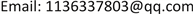Received: Feb. 1st, 2019; accepted: Feb. 18th, 2019; published: Feb. 25th, 2019ABSTRACT

Smart grid metering system has a large amount of electric power data. It can improve electric power efficiency and user experience by processing data reasonably and efficiently and making full use of data to improve the business of metering system on the user side. The edge computing model migrates all or part of the computing tasks of the application server from the cloud to the edge device on the edge of the network, thus greatly improving data transmission efficiency, and ensuring the real-time performance of data processing, while reducing the possibility of network congestion. In this paper, a method of electricity forecasting based on edge computing under metering system is proposed. This method is based on the excellent real-time performance of the edge computing model, combined with the gradient boosting decision tree algorithm and the online learning method in the machine learning field. It can efficiently and accurately perform real-time training and electricity forecasting on massive power data.

Keywords:Metering System, Edge Computing, Gradient Boosting Decision, Online Learning

1电子科技大学通信抗干扰国家级重点实验室，四川 成都

2南方电网科学研究院有限责任公司，广东 广州Copyright © 2019 by author(s) and Hans Publishers Inc.1. 引言

2. 系统模型

2.1. 边缘计算

2.2. 基于边缘计算的电量预测模型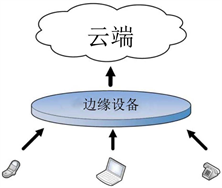Figure 1. Edge computing model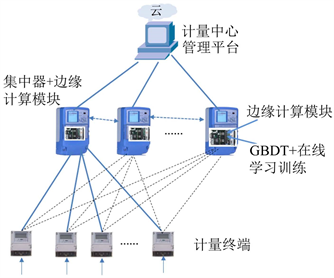Figure 2. Electricity forecasting model based on edge computing

3. 基于梯度提升树的电量预测方法

3.1. 在线学习与离线学习

3.2. 梯度提升树学习方法

$L\left(y,{f}_{m-1}\left({x}_{i}\right)\right)$ (1)

${f}_{m}\left({x}_{i}\right)={f}_{m-1}\left({x}_{i}\right)-\alpha \frac{\partial L\left(y,{f}_{m-1}\left({x}_{i}\right)\right)}{\partial {f}_{m-1}\left({x}_{i}\right)}$ (2)

${f}_{m}\left({x}_{i}\right)={f}_{m-1}\left({x}_{i}\right)+T\left({x}_{i};{\Theta }_{m}\right)$ (3)

$T\left({x}_{i};{\Theta }_{m}\right)=-\frac{\partial L\left(y,{f}_{m-1}\left({x}_{i}\right)\right)}{\partial {f}_{m-1}\left({x}_{i}\right)}$ (4)

$-{\left[\frac{\partial L\left(y,f\left({x}_{i}\right)\right)}{\partial f\left({x}_{i}\right)}\right]}_{f\left(x\right)={f}_{m-1}\left(x\right)}$ (5)

$T=\left\{\left({x}_{1},{y}_{1}\right),\left({x}_{2},{y}_{2}\right),\cdots ,\left({x}_{t},{y}_{t}\right)\right\}$ (6)

${f}_{0}\left(x\right)=\mathrm{arg}\underset{c}{\mathrm{min}}\underset{i=1}{\overset{t}{\sum }}L\left({y}_{i},c\right)$ (7)

a：对每个时刻 $i=1,2,\cdots ,t$ 的电力数据 $\left({x}_{i},{y}_{i}\right)$ ，计算当前模型负梯度：

${r}_{mi}=-\frac{\partial L\left({y}_{i},{f}_{m-1}\left({x}_{i}\right)\right)}{\partial {f}_{m-1}\left({x}_{i}\right)}$ (8)

b：对 ${r}_{mi}$ 拟合一个CART树，得到第 $m$ 棵树的叶节点区域 ${R}_{mj}$$j=1,2,\cdots ,J$ 。这些叶节点区域 ${R}_{mj}$ 会将训练数据集 $T$ 分为 $J$ 份。

c：对 $j=1,2,\cdots ,J$ ，计算：

${c}_{mj}=\mathrm{arg}\underset{c}{\mathrm{min}}\underset{{x}_{i}\in {R}_{mj}}{\sum }L\left({y}_{i},{f}_{m-1}\left({x}_{i}\right)+c\right)$ (9)

${c}_{mj}$ 即为能使当前模型的损失函数达到极小值的第 $m$ 棵CART树的叶节点区域 ${R}_{mj}$ 对应的输出。

d：更新模型：

${f}_{m}\left(x\right)={f}_{m-1}\left(x\right)+\underset{j=1}{\overset{J}{\sum }}{c}_{mj}I\left(x\in {R}_{mj}\right)$ (10)

${f}_{M}\left(x\right)=\underset{m=1}{\overset{M}{\sum }}\underset{j=1}{\overset{J}{\sum }}{c}_{mj}I\left(x\in {R}_{mj}\right)$ (11)

${\stackrel{^}{y}}_{t+1}={f}_{M}\left({x}_{t+1}\right)=\underset{m=1}{\overset{M}{\sum }}\underset{j=1}{\overset{J}{\sum }}{c}_{mj}I\left({x}_{t+1}\in {R}_{mj}\right)$ (12)

3.3. 基于边缘计算的电量预测流程

4. 实验验证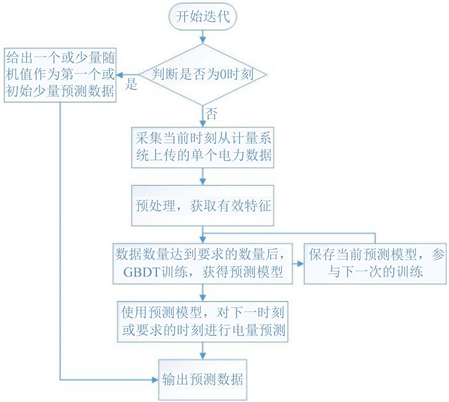Figure 3. GBDT and online learning training process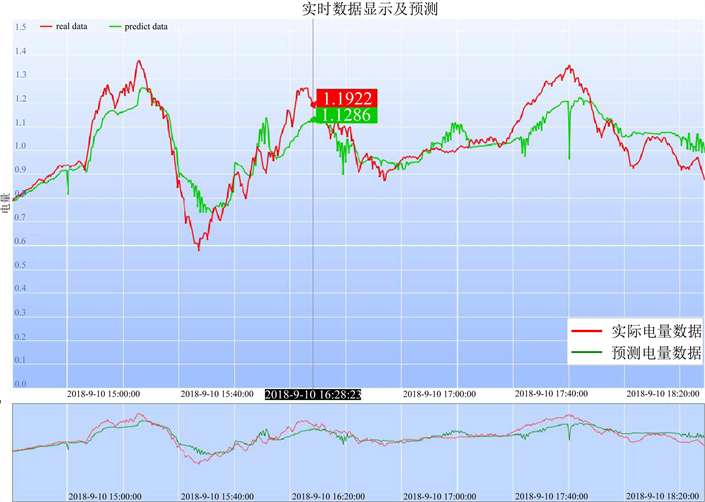Figure 4. One-time prediction effect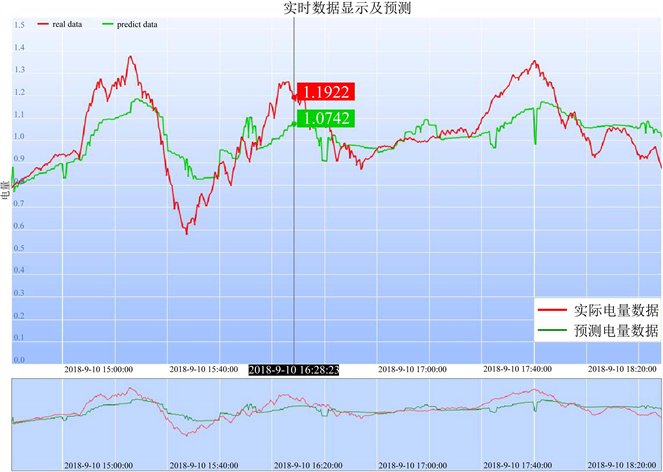Figure 5. Three-time prediction effect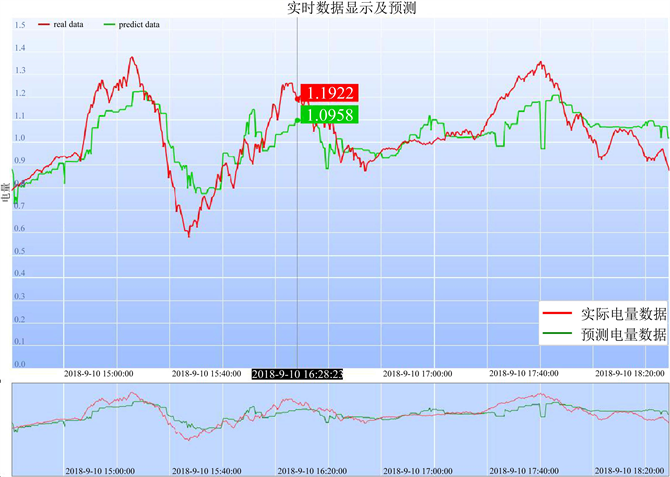Figure 6. Five-time prediction effect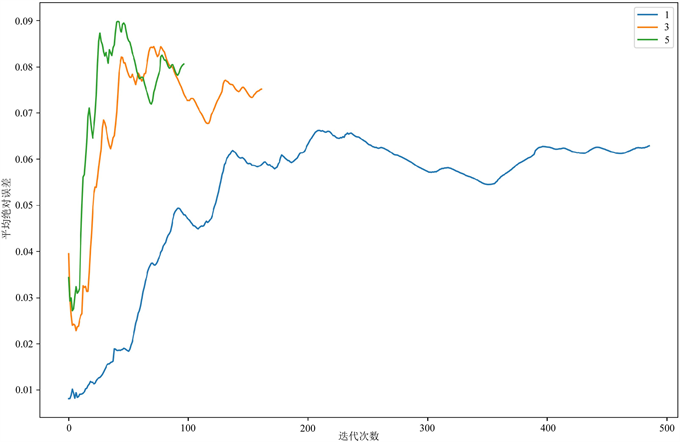Figure 7. Average absolute error of different forecast times

5. 总结

Electricity Forecasting Based on Edge Computing under Metering System[J]. 智能电网, 2019, 09(01): 23-30. https://doi.org/10.12677/SG.2019.91003

1. 1. 余贻鑫, 栾文鹏. 智能电网[J]. 电网与清洁能源, 2009, 25(1): 7-11.

2. 2. 巫志平. 电能量计量系统地县一体功能需求分析与研究[J]. 智能电网, 2(5): 49-54.

3. 3. 施巍松, 孙辉, 曹杰, 等. 边缘计算: 万物互联时代新型计算模型[J]. 计算机研究与发展, 2017, 54(5): 907-924.

4. 4. Sousa, J.C., Neves, L.P. and Jorge, H.M. (2012) Assessing the Relevance of Load Profiling Information in Electrical Load Forecasting Based on Neural Network Models. International Journal of Electrical Power and Energy Systems, 40, 85-93.
https://doi.org/10.1016/j.ijepes.2012.02.008

5. 5. Friedman, J.H. (2001) Greedy Function Approximation: A Gradient Boosting Machine. The Annals of Statistics, 29, 1189-1232.
https://doi.org/10.1214/aos/1013203450

6. 6. Santos, P.J., Martins, A.G. and Pires, A.J. (2007) Designing the Input Vector to ANN-Based Models for Short-Term Load Forecast in Electricity Distribution Systems. International Journal of Electrical Power and Energy Systems, 29, 338-347.
https://doi.org/10.1016/j.ijepes.2006.09.002

7. 7. Duan, Q.H., Liu, J.R. and Zhao, D.F. (2017) Short Term Electric Load Forecasting Using an Automated System of Model Choice. International Journal of Electrical Power and Energy Systems, 91, 92-100.
https://doi.org/10.1016/j.ijepes.2017.03.006